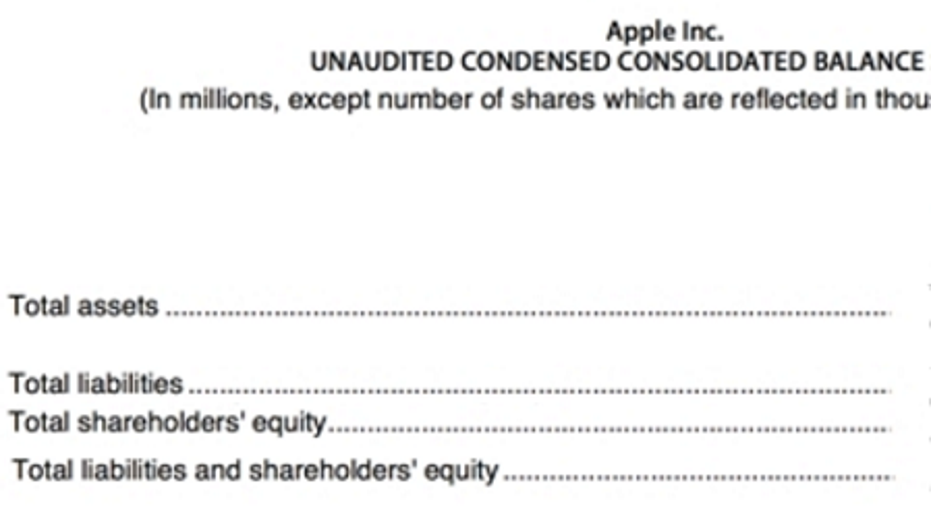# How to Calculate the Debt Ratio Using the Equity MultiplierWhen you want to get an idea of a company's financial condition, ratio analysis is one of the tools of the trade. In the following article, you'll learn about two useful balance sheet ratios: the debt ratio and the equity multiplier, and you'll learn the relationship between the two and how to calculate one using the other.

Companies finance their assets through two means: Debt and equity. Let's imagine company A has assets totaling \$300,000 that is has financed issuing \$200,000 worth of debt and \$100,000 of equity:

Calculating the debt ratioThe debt ratio is the proportion of a company's assets that is financed through debt:

Debt ratio = Total debt / Total assets

The more debt the company carries relative to the size of its balance sheet, the higher the debt ratio. Total debt cannot be negative, nor can it be greater than total assets (ignoring cases of negative equity), therefore the debt ratio must be between 0% and 100% (the debt ratio is commonly expressed as a percentage).

In the case of company A, we obtain:

Debt ratio = ( \$200,000 / \$300,000 ) = 2/3 67%

Two-thirds of the company A's assets are financed through debt, with the remainder financed through equity.

Calculating the equity multiplierThe equity multiplier, on the other hand, relates the size of the balance sheet (i.e. total assets) to the amount of equity ; in other words, it measures the factor by which the company's equity has been leveraged:

Equity multiplier = Total assets / Total equity

The greater the equity multiplier, the higher the amount of leverage.

For company A, we obtain:

Equity multiplier = ( \$300,000 / \$100,000 ) = 3.0 times

How to calculate the debt ratio using the equity multiplier (and vice-versa)The debt ratio and the equity multiplier are linked by the following formula:

Debt ratio = 1- ( 1 / Equity multiplier )

Let's verify the formula for company A:

Debt ratio = 1-( 1 / 3 ) = 2 / 3 67%, which is exactly the result we found above.

If you want to know how the formula linking the debt ratio was derived, it's very straightforward using some basic algebra. If you're interested, you can find the derivation at the bottom of the article.

Examples: Apple Inc. and Cheseapeake Energy Corporation

Below is the relevant balance sheet data taken straight from Apple Inc's most recent quarterly report:

Source: Apple, press release

Thus:

Apple

Equity multiplier

\$293,284/ \$128,267 = 2.29 x

Debt ratio

\$165,017/ \$293,284 = 56.3%

Given the size of the operating cash flows Apple generates and the quality of its business, Apple's use of debt is conservative and its equity multiplier reflect this.

Next, we have Chesapeake Energy's condensed balance sheet, taken from its most recent quarterly report:

Source: Chesapeake Energy Corporation press release

We're ready to run the numbers:

Apple

Chesapeake Energy

Equity multiplier

2.29 x

\$17,357/ \$\$2,397 = 7.2 x

Debt ratio

56.3%

\$14,960/ \$17,357 = 86.2%

Chesapeake Energy is no Apple! Its higher ratios reflect a very significant use of debt, and given Chesapeake Energy's exposure to commodities prices, this is a very different proposition in terms of the business' financial risk.

Extra credit: Deriving the equation linking the debt ratio and the equity multiplier:

Equity multiplier = Total assets / Total equity

Another way of writing that equation is:

Total equity / Total assets = ( 1 / Equity multiplier ) (1)

According to the fundamental equation of accounting:

Total equity = Total assets-Total debt

If we substitute that into equation (1), we obtain:

( Total assets-Total debt ) / Total assets = ( 1 / Equity multiplier )

Which simplifies to:

1- ( Total debt / Total assets ) = ( 1 / Equity multiplier )

Re-arranging the terms:

( Total debt / Total assets ) = 1- ( 1 / Equity multiplier )

But ( Total debt/ Total assets ) is nothing other than the debt ratio, so we have our result:

Debt ratio = 1- ( 1 / Equity multiplier )

Voila!

This article is part of The Motley Fool's Knowledge Center, which was created based on the collected wisdom of a fantastic community of investors. We'd love to hear your questions, thoughts, and opinions on the Knowledge Center in general or this page in particular. Your input will help us help the world invest, better! Email us atknowledgecenter@fool.com. Thanks -- and Fool on!

The article How to Calculate the Debt Ratio Using the Equity Multiplier originally appeared on Fool.com.

The Motley Fool owns shares of and recommends Apple. Try any of our Foolish newsletter services free for 30 days. We Fools may not all hold the same opinions, but we all believe that considering a diverse range of insights makes us better investors. The Motley Fool has a disclosure policy.